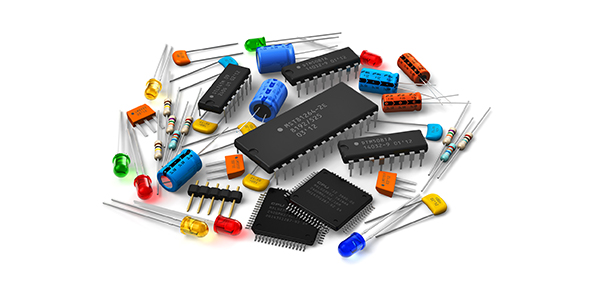10 Questions | Total Attempts: 1520SettingsLet us help each other, fellow ECE reviewees and students. Do not result to such lowly behavior. As they say, a rising tide floats all boats. Lets mind our manners. Respect, engineers. Good for testing your knowledge and mastery on different review materials. Question bank inlcudes Malvino

• 1.
An ideal voltage source has
• A.

Zero internal resistance

• B.

Infinite internal resistance

• C.

• D.

• 2.
A real voltage source has
• A.

Zero internal resistance

• B.

Infinite internal resistance

• C.

A small internal resistance

• D.

A large internal resistance

• 3.
The net charge of a neutral copper atom is
• A.

0

• B.

+1

• C.

- 1

• D.

+4

• 4.
At room temperature an intrinsic silicon crystal acts approximately like
• A.

Battery

• B.

Conductor

• C.

Insulator

• D.

Piece of copper wire

• 5.
What causes the depletion layer?
• A.

Doping

• B.

Recombination

• C.

Barrier Potential

• D.

Ions

• 6.
With a half-wave rectified voltage across the load resistor, load current flows for what part of a cycle?
• A.

0 degrees

• B.

90 degrees

• C.

180 degrees

• D.

360 degrees

• 7.
The load voltage is approximately constant when a zener diode is
• A.

Forward-biased

• B.

Reverse-biased

• C.

Operating in the breakdown region

• D.

Unbiased

• 8.
For typical operation, you need to use reverse bias with a
• A.

Zener diode

• B.

Photodiode

• C.

Varactor

• D.

All of the above

• 9.
. If the depletion layer gets wider, the capacitance
• A.

Decreases

• B.

Stays the same

• C.

Increases

• D.

Is variable

• 10.
The currents of a pnp transistor are
• A.

Usually smaller than npn currents

• B.

Opposite npn currents

• C.

Usually larger than npn currents

• D.

Negative

Related TopicsBack to top